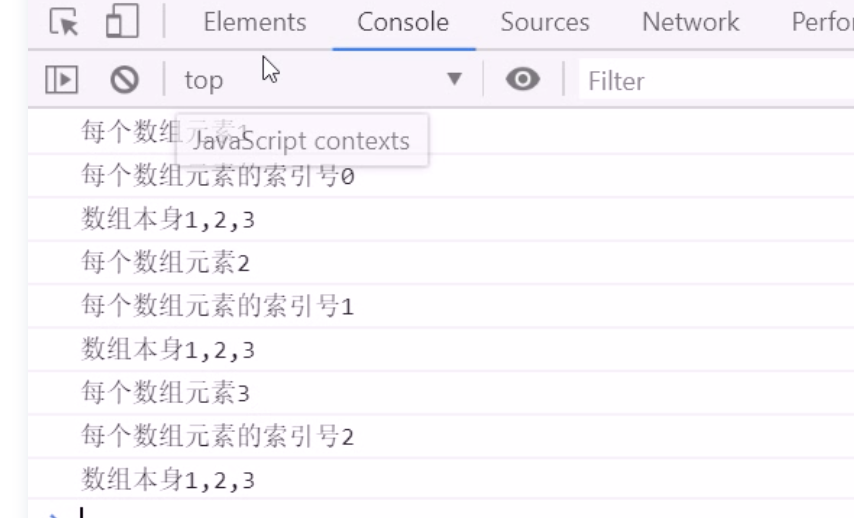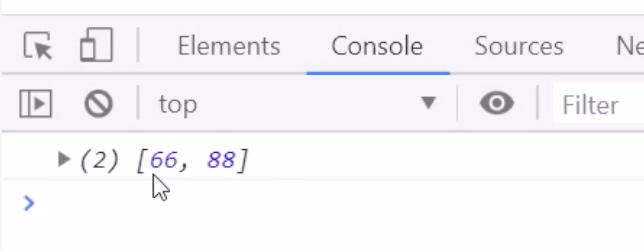# 带你学习ES5中新增的方法

2020-11-06 20:37:29 叫我詹躲躲

• 数组方法
• 字符串方法
• 对象方法

### 2. 数组方法

#### 2.1 forEach跟jQuery的each用法类似。语法是：

``array.forEach(function(currentValue, index, arr))``
• currentValue: 数组当前项的值
• index: 数组当前项的索引
• arr: 数组对象本身
``````var arr = [1,2,3]
arr.forEach(function(value, index, array){

console.log('每个数组元素'+ value)
console.log('每个数组元素的索引值'+ index)
console.log('数组本身'+ array)
})``````#### 2.2 map()

``array.map(function(currentValue, index, arr))``

map() 方法创建一个新数组，其结果是该数组中的每个元素都调用一个提供的函数后返回的结果。

• currentValue: 数组当前项的值
• index: 数组当前项的索引
• arr: 数组对象本身
``````var array1 = [1, 4, 9, 16];
var map1 = array1.map(function(value, index, arr) {

return value * 2
});
console.log(map1) //[2,8,18,32]``````

#### 2.3 filter()

``array.filter(function(currentValue, index, arr))``

filter()方法用于筛选创建一个新的数组，新数组中的元素是通过检查指定数组中符合条件的所有元素，主要用于筛选数组。
filter()方法是直接返回一个新的数组

• currentValue: 数组当前项的值
• index: 数组当前项的索引
• arr: 数组对象本身
``````var arr = [12,66,88]
// 选出大于20的
var newarr = arr.filter(function(value, index){

return value >=20
})

console.log(newArr) //[66, 88]``````#### 2.4 some()

``array.some(function(currentValue, index, arr))``

some()方法用于检测数组中的元素是否满足指定条件，通俗点查找数组中是否有满足条件的元素

• currentValue: 数组当前项的值
• index: 数组当前项的索引
• arr: 数组对象本身
``````var arr=[10,30,4]
var b = arr.some(function(value){

return value > 20
})
console.log(b) //true``````

#### 2.5 every()

``array.every(function(currentValue, index, arr))``

every() 方法测试一个数组内的所有元素是否都能通过某个指定函数的测试。它返回一个布尔值。

• currentValue: 数组当前项的值
• index: 数组当前项的索引
• arr: 数组对象本身

#### 2.6 isArray()

``Array.isArray(obj)``

Array.isArray() 用于确定传递的值是否是一个 Array

• obj是需要检测的值。如果是数组则返回true,否则返回false

1. filter 是查找满足条件的元素，返回的是一个数组，而且是把所有满足条件的元素返回回来
2. some 是查找满足条件的元素是否存在，返回的是一个布尔值，如果查找第一个满足条件的元素，就终止循环。如果数组中查询唯一个元素，用some方法更为合适，因为它找到这个元素，就不在进行循环，效率更高
3. every是查找所有的元素是否符合条件，返回的是一个布尔值
4. map是创建一个新数组，其结果是该数组中的每个元素都调用一个提供的函数后返回的结果

### 3. 字符串方法

trim() 方法会从一个字符串的两端删除空白字符

``str.trim()``

trim() 方法并不影响原字符串本身，它返回的是一个新字符串。

### 4. 对象方法

Object.defineProperty()定义对象中新增属性或修改原有的属性

``Object.defineProperty(obj, prop, descriptor)``
• obj: 必需。目标对象
• prop: 必需。 需定义或修改的属性名字
• descriptor: 必需。目标属性所拥有的特性

Object.defineProperty() 第三个参数descriptor说明： 以对象形式{}书写

• value：设置属性的值，默认为undefined
• writable:值是否可以重写。true | false 默认为false
• enumerable: 目标属性是否可以被枚举。true | false 默认为false
• configurable: 目标属性是否可以被删除或是否可以再次修改特性true | false 默认为false
``````var obj = {

id: 1,
pname: '小米',
price: 1999
}
//以前的对象添加和修改属性的方式
obj.num = 1000
obj.pirce = 99
console.log(obj)

//Object.deefineProperty()定义新属性或者修改原有的属性
Object.defineProperty(obj, 'num',{

value: 1000,
})
Object.defineProperty(obj, 'pricee',{

value: 9.9,
})
Object.defineProperty(obj, 'id',{

//如果值为false,则不允许修改
writable: false,
})

value: '中国郑州市',
//如果值为false,则不允许遍历，枚举
enumerable: false
})
console.log(obj)
console.log(Object.keys(obj))``````

### 总结

https://my.oschina.net/u/3995971/blog/4558944